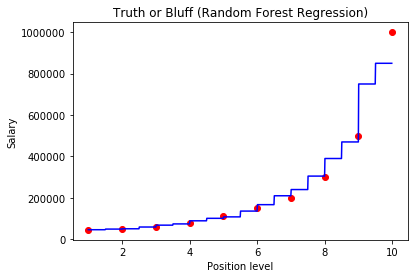0%

Random forest is a non linear regression algorithm that creates decision trees on data samples and then gets the prediction from each of them and finally selects the best solution by majority vote.
*It is a type of ensemble learning that means combining many models into one and here it combines many decision trees.

### Here is how Random Forest looks like:#### Now Let us start

For this model we will be using random forest to predict a salary of an employee with position level of 6.5

## Importing the libraries

In :
import numpy as np
import matplotlib.pyplot as plt
import pandas as pd


## Importing the dataset / Create Own

In :
df = pd.DataFrame({'Position': ['Business Analyst', 'Junior Consultant', 'Senior Consultant', 'Manager', 'Country Manager', 'Region Manager', 'Partner', 'Senior Partner', 'c-level', 'CEO'], 'Level':[1,2,3,4,5,6,7,8,9,10], 'Salary': [45000, 50000, 60000, 80000, 110000, 150000, 200000, 300000, 500000, 1000000]})
x = df.iloc[: , 1:2].values
y = df.iloc[:, 2].values
print(df)

            Position  Level   Salary
1  Junior Consultant      2    50000
2  Senior Consultant      3    60000
3            Manager      4    80000
4    Country Manager      5   110000
5     Region Manager      6   150000
6            Partner      7   200000
7     Senior Partner      8   300000
8            c-level      9   500000
9                CEO     10  1000000


## Fitting Random Forest Regression to the dataset

In :
from sklearn.ensemble import RandomForestRegressor
regressor = RandomForestRegressor(n_estimators = 10, random_state = 0)
regressor.fit(x, y)

Out:
RandomForestRegressor(bootstrap=True, criterion='mse', max_depth=None,
max_features='auto', max_leaf_nodes=None,
min_impurity_decrease=0.0, min_impurity_split=None,
min_samples_leaf=1, min_samples_split=2,
min_weight_fraction_leaf=0.0, n_estimators=10, n_jobs=None,
oob_score=False, random_state=0, verbose=0, warm_start=False)

## Predicting a new result

In :
y_pred = regressor.predict([[6.5]])


## Visualising the Random Forest Regression results (higher resolution)

In :
X_grid = np.arange(min(x), max(x), 0.01)
X_grid = X_grid.reshape((len(X_grid), 1))
plt.scatter(x, y, color = 'red')
plt.plot(X_grid, regressor.predict(X_grid), color = 'blue')
plt.title('Truth or Bluff (Random Forest Regression)')
plt.xlabel('Position level')
plt.ylabel('Salary')
plt.show()In [ ]: